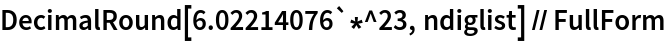#Function Repository Resource:

# DecimalRound

Round a machine number to a prescribed number of significant digits using conventional rounding rules

Contributed by: Peter Valko  |  Peter Valko
 ResourceFunction["DecimalRound"][machnum,ndig] rounds the machine number machnum to ndig significant digits using conventional rounding rules.

## Details and Options

The argument ndig should be an integer between 1 and 15. If not, it is rounded and clipped.
The default value of ndig is 6.
ResourceFunction["DecimalRound"] has the Listable attribute, so either argument can be a list. However, both arguments cannot be lists simultaneously.
In case of failure, the first argument is returned.

## Examples

### Basic Examples (3)

Create a machine number approximating π:

 In:=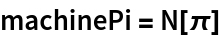Out=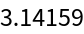Use DecimalRound to round the machine number to four significant digits:

 In:=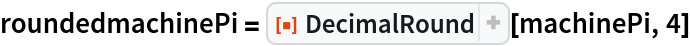Out=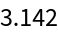Using InputForm shows that these are different values:

 In:=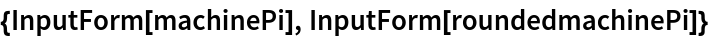Out=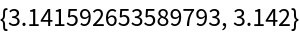### Applications (3)

Generate a random matrix with elements drawn from the stable probability distribution, calculate the matrix inverse, check the product and compute its matrix norm:

 In:=Make a version of the matrix rounded to six significant digits and process as before:

 In:=Compare the results:

 In:=Out=In:=Out=The product norm should be near 1. We can see that rounding to 6 significant digits before inversion gives rise to a discernable change in the computed inverse.

### Properties and Relations (2)

Compare to Round:

 In:=In:=Out=As the results are not machine numbers, we make them so:

 In:=In:=Out=A trailing 0000x or 9999x is not desirable. Moreover the approach works only for numbers of order one, so now scale the second argument:

 In:=In:=Out=Use of RealDigits shows similar issues:

 In:=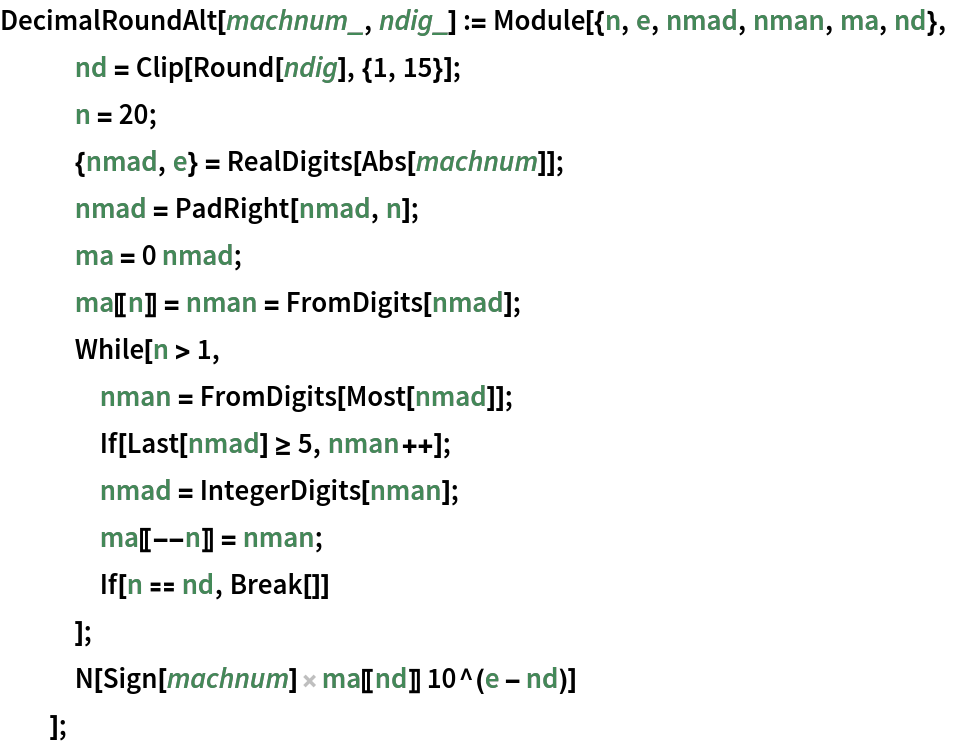In:=Out=### Possible Issues (2)

In case of any error, the first argument is returned. Here the first argument is not a machine number:

 In:=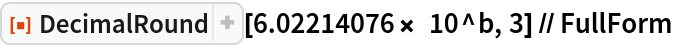Out=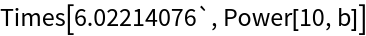The first argument is returned unevaluated here as well:

 In:=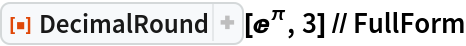Out=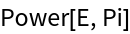## Author Notes

In general, the precision of an approximate real number is the effective number of decimal digits in it which are treated as significant for computations. Machine numbers – in contrast to the other type of approximate numbers, the arbitrary‐precision numbers – contain a fixed number of digits (MachinePrecision). Since they maintain no information on their precision, all their digits are treated as significant during computations. In rare occasions one wants to prescribe the effective number of digits in a machine number, putting zeros into the trail and perhaps modifying the last significant digit. In everyday life, this operation is called rounding, but the Wolfram Language function Round is not designed specifically for machine numbers and it is not straightforward how to use it to set explicitly the number of significant digits in the decimal representation of a machine number. The resource function DecimalRound takes a machine number and the number of required digits and attempts to provide the result as a machine number with exactly the requested number of significant digits.

In the following example, numlist consists of five numbers, originally having wildly spread precisions. For instance, the first number is the AvogadroConstant in SI which is perfectly defined by an international standard. On the other hand, the fourth number is the result of a calculation to approximate π via dividing 22 by 7 and the indicated precision is itself the result of an approximate calculation. Here is the list:

 In:=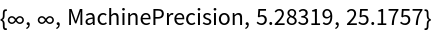When we create the list of machine numbers, we lose some information on precision (for instance our approximate π will look like a 16 digit good approximation), but computations and storage will be more efficient in the world of machine numbers:

 In:=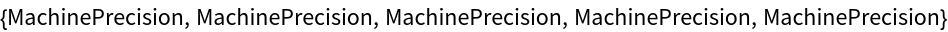Interestingly, some information is preserved, for instance the Avogadro number will still show that it has nine “good” digits, albeit only implicitly, by the fact that seven trailing digits are zeros. If we want to convey our knowledge regarding the approximate π , we should round it to – say – 3 digits, as we would do it in a hand calculation. DecimalRound can do this. To be more general, in this example we create a variable which contains possible requirements for the number of significant digits:

 In:=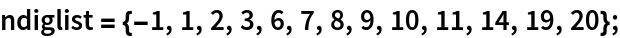Then DecimalRound is called with various combinations of the two arguments:

 In:=In the output the column heading shows the rounded machine numbers. The row heading shows the requested digit counts. We use FullForm to see what we are really having in the result matrix:

 In:=Since the function is Listable, we can easily get a whole row of the matrix:

 In:=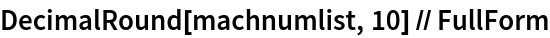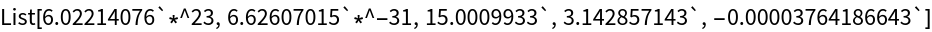as well as as a whole column:

 In:=